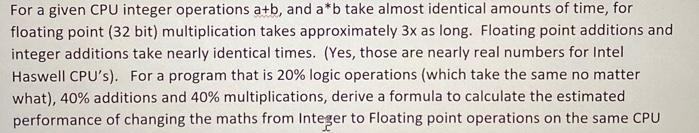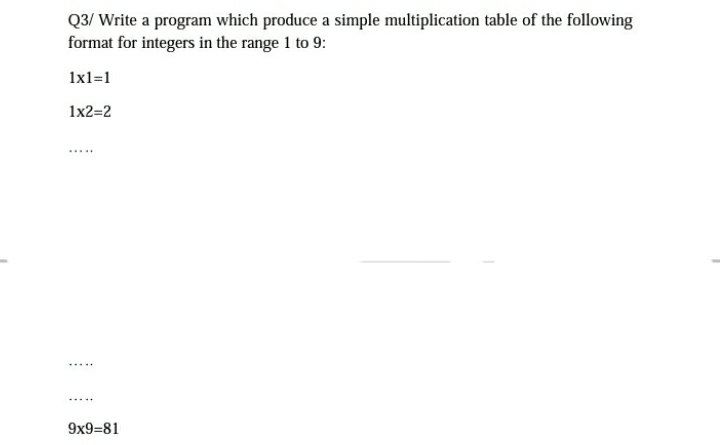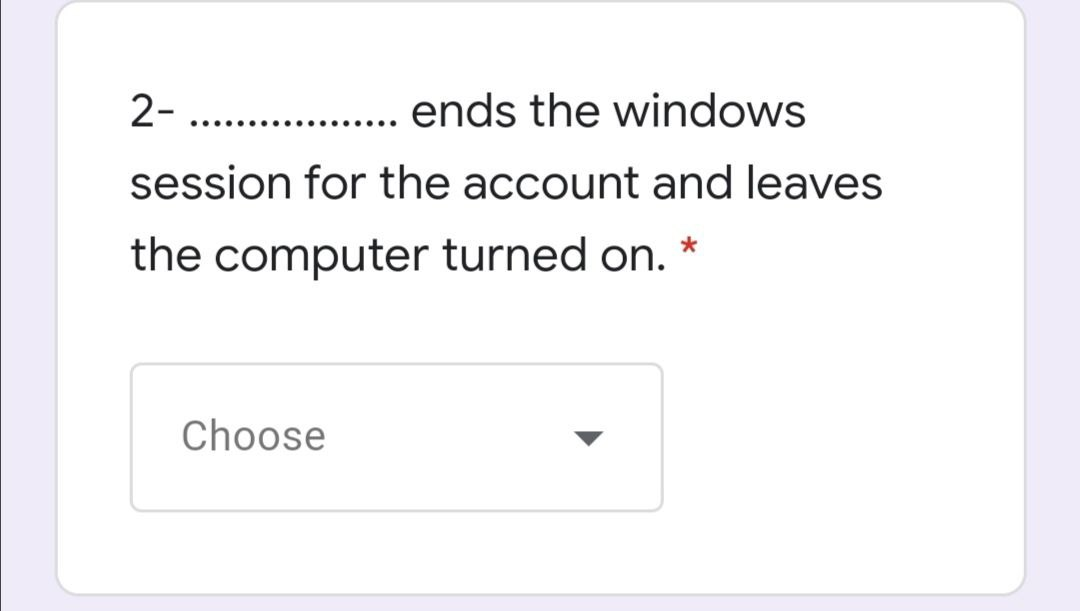## (Solved):Q: Make the functio…

Question

Make the functions below according to the attached class diagram and GUI program mockup.

 No The function to be made 1 Create Transaction Order_ID = 1 Order_Date = 11-2-2021 Customer_ID = 2 Customer_RefNo = 001 / I / S / 2021   When the TextField ‘txtCustomerID’ is filled and we click the ‘View’ Button, then the TextField ‘txtViewCustomerName’ will display the ‘Customer_Name’ field from the Customer’s Table according to the input Customer_ID. If the data is not found, a notification will appear “Customer not registered”. 2 Fill in the List Combo Box Product_ID by retrieving data from the Product Fied Table Product_ID 3 If the button ‘Tambah Item’ is clicked, then data from Product_ID, txtProduct_Name, txtQuantity, txtPrice will be saved to the ‘Order Detail’ Tabke (according to the mockup), then also enter the Sub Total field in the ‘Order Detail’ table with the calculation of txtQuantity * txtPrice. 4 When the ‘Hapus’ button is clicked, data from Product_ID, txtProduct_Name, txtQuantity, txtPrice will be deleted. 5 Display txtGrandTotal based on Sum of Subtotals from ‘Order Detail’ Table. 6 Create Order Details with Item 1: Product_ID = 2, Quantity = 4, Price = 11,000 Item 2: Product_ID = 4, Quantity = 2, Price = 21,000   If you press the ‘Simpan’ button, the data will be saved to the Order and Order_Detail Tables and stock updates to the product table, with the following mechanism:   Order: Order_ID = txtOrder_ID, Order_Date = txtOrder_Date, Customer_ID = txtCustomer_ID, Customer_RefNo = txtCustomer_RefNo   Order_Detail: Order_ID = txtOrder_ID, Product_ID = ID From the Order Details Table, Quantity = Quantity from the Order Details Table, Price = Price from the Order Details Table   Product: Update Stock added Quantity according to the same Item Code. If there are 2 items in the Order Detail Table, you must update the stock to the 2 items. By calculating Stock = Stock – Quantity. For proof, attach the output stock to the product table before and after the transaction. 7 If the ‘Keluar’ button is clicked it will exit the application

Submit Answers in the form of Java Coding.

## (Solved):Q: Which bit in the…

Question

1. Which bit in the PTE does the operating system use for approximating LRU replacement?

## (Solved):Q: Artificial Intel…

Question

Artificial Intelligence.

Use the A* search strategy

## (Solved):Q: Write a C++ prog…

Question
Write a C++ program to declare a class Rectangle with two data members length and width and two function members:
1) to set the values of length and width (this function should be defined outside the class).
2) to calculate area (this function should be defined inside the class).

## (Solved):Q: Write python cod…

Question
Write python code to calculate A. O. C?

## (Solved):Q: For a given CPU …

Questionhelp_outline close For a given CPU integer operations a+b, and å b take almost Ical amount floating point (32 bit) multiplication takes approximately 3x as long. Floating point additions and integer additions take nearly identical times. (Yes, those are nearly real numbers for Intel Haswell CPU’s). For a program that is 20% logic operations (which take the same no matter what), 40% additions and 40% multiplications, derive a formula to calculate the estimated performance of changing the maths from Integer to Floating point operations on the same CPU fullscreen

## (Solved):Q: Q3/ Write a prog…

Questionhelp_outline close Q3/ Write a program which produce a simple multiplication table of the following format for integers in the range 1 to 9: 1×1=1 1×2=2 9×9=81 fullscreen

## (Solved):Q: 1. Supposed a ba…

Question

1. Supposed a ball is thrown straight up in the air with an initial velocity of 50 feet per second and an initial height of 5 feet. How high will the ball be after 3 seconds? Note: The height after t seconds is given by the expression – 16t+vot+ho, where ve is the initial velocity and he is the initial height.

## (Solved):Q: 2- ends the wind…

Questionhelp_outline close 2- ends the windows . session for the account and leaves the computer turned on. * Choose fullscreen

Question
OUTPUT 1